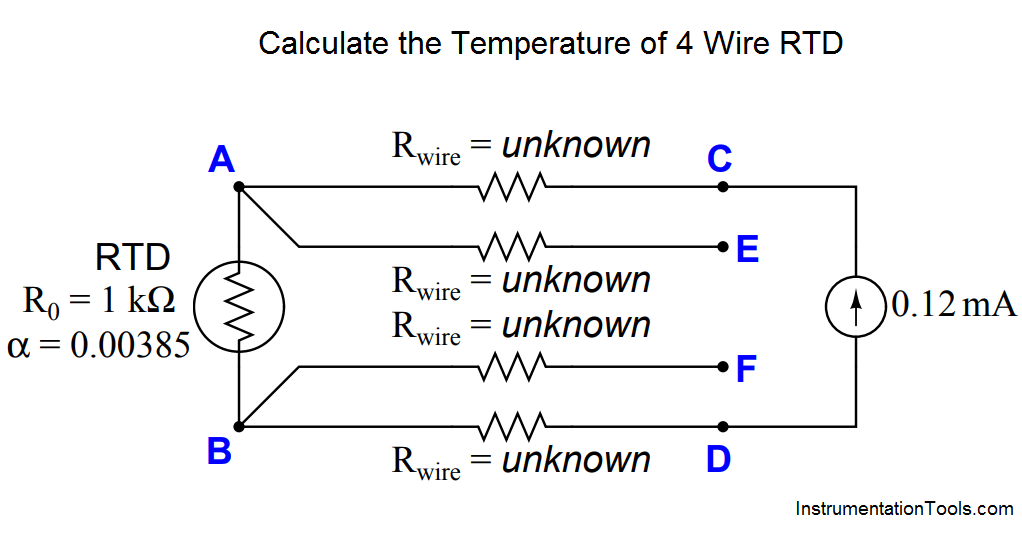# Calculate the Temperature of 4 Wire RTD

Determine the temperature of the RTD, given VCD = 140.5 millivolts and VEF = 140.1 millivolts:Assume a 1 kΩ RTD with α = 0.00385, and all wire resistances completely unknown (not assumed to be equal).

VRTD = 140.1 mV

RRTD = 1167.5 Ω

T = 43.5 oC = 110.31 oF (both values calculated from formula, not table)

1.